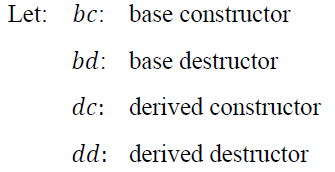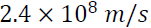1. Which one of the following is an error reporting protocol?

(a) ARP
(b) TCP
(c) ICMP
(d) UDP

2. What is the probability of getting a number greater than 4 while single throw of a dice?

(a) 1/4
(b) 2/3
(c) 1/2
(d) 1/3

3. Three sections of a class have respectively 25,50,25 students. The mean marks obtained by the first two sections are respectively 60 and 55. The overall mean of all three sections is 58. The mean of the third section is

(a) 52
(b) 57
(c) 58
(d) 62

4. A shop keeper sold product A at Rs. 266 after giving a discount of 5 % on the marked price. Without this discount he could have earned a profit of 12 % on the cost price. The cost price of A is

(a) 200
(b) 227
(c) 250
(d) 275

5. Let 𝐵 be base class and 𝐷 be derived class in 𝐶 ++.When an object of derived class is created and then deleted, the correct sequence of invocations is:
(a) bc, bd, dc, dd
(b) dc, bc, dd, bd
(c) bc, dc, dd, bd
(d) dc, dd, bc, bd

6. The performance of the network is often evaluated by which of the following two networking metrics?

(a) Speedup and accuracy
(b) Throughput and delay
(c) Speedup and delay
(d) Throughput and accuracy

7. A sine wave is offset 1/6 cycle with respect to time 0. Its phase will be nearly:

8. If a periodic signal is decomposed into 5 sine waves with frequencies of 100 Hz, 300 Hz, 500 Hz, 700 Hz and 900 Hz, its bandwidth will be:

(a) 800 Hz
(b) 700 Hz
(c) 600 Hz
(d) 500 Hz

9. A network with bandwidth of 10 Mbps can pass only an average of 12,000 frames per minute with each frame carrying an average of 10,000 bits. The throughput of this network will be:

(a) 2 Mbps
(b) 4 Mbps
(c) 6 Mbps
(d) 8 Mbps

10. Assume the distance between the sender and the receiver is 12,000 km , the bandwidth of the network is 1 Mbps and that light travels at. The propagation time and the transmission time to transmit 5-MB message (an image), will be respectively: